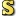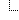﻿ComplexMatrix Class
Cove - A Practical Quantum Computer Programming Framework
ComplexMatrix Class
NamespacesCove.ClassicalUtilitiesComplexMatrix
A matrix class of size m x n that contains complex numbers.Declaration Syntax
 C# Visual Basic Visual C++
`public class ComplexMatrix : ICoveObject`
```Public Class ComplexMatrix _
Implements ICoveObject```
`public ref class ComplexMatrix : ICoveObject`Members
 All Members Constructors Methods Fields PublicProtected InstanceStatic DeclaredInherited
IconMemberDescriptionComplexMatrix()()()
Default constructor, construct a 2 x 2 matrix with all entries set to 0.ComplexMatrix(Int32, Int32)
Construct a Rows x Columns sized matrix. Every entry will be 0ComplexMatrix(array<Complex,2>[,](,)[,])
Construct a matrix based on the specified cellsComplexMatrix(Int32)
Construct an identity matrix or size IdentitySize. The Identity matrix created is IdentitySize x IdentitySize in size. Along the diagonal is 1, all other entries are 0.Addition(ComplexMatrix, ComplexMatrix)
Perform addition between two matrices of equal sizeAjoint()()()
Get the Ajoint (Hermitian conjugate) of this matrix. This is typically notated by A^(Cross)caCells
The individual cells of the matrixClearAllCells()()()
Clear all the cells in the matrix to (0 + 0i)Clone()()()
Returns a deep copy of this matrix, so the original can be modified without affecting the copy. (As would happen in copy by reference.)ConjugateTranspose()()()
Return the conjugate transpose of this matrix. This is typically written A^H, although sometimes A^* is used.CopyFrom(ComplexMatrix)
Set this instance to a deep copy of Source. (Clone returns a deep copy of this object, while CopyFrom sets this object to a deep copy.CreateIdentityMatrix(Int32)
Get the identity matrix (square matrix) of the specified Length. Hence the returned matrix is a Length x Length matrix with 1's along the diagonal.Equality(ComplexMatrix, Int32)
Equality operator for a complex matrix and an int.Equality(ComplexMatrix, Double)
Equality operator for a ComplexMatrix and a double.Equality(ComplexMatrix, ComplexMatrix)
Equality operator for two ComplexMatrix instancesEquality(ComplexMatrix, Complex)
Equality operator to a ComplexMatrix and a complexEquals(ComplexMatrix)
Are two matrices equal?Equals(Object)
Handle equality against any object.
(Overrides Object.Equals(Object).)Equals(Int32)
A complex matrix can only be equal to an int if it is a 1 x 1 matrix of the same value of an int. This allows for multiplication to easily be checked out as in:  [7 -4 5] = 8Equals(Double)
A complex matrix can only be equal to a double if it is a 1 x 1 matrix of the same value of a double. This allows for multiplication to easily be checked out as in:  [7 -4 5] = 8Equals(Complex)
A complex matrix can only be equal to a complex if it is a 1 x 1 matrix of the same value of a double. This allows for multiplication to easily be checked out as in: [(3 + 0i)] [(7 + 0i) (-4 + 0i) (5 + 0i)][(2 + 0i)] = (8 + 0i) [(1 + 0i)]Finalize()()()
Allows an Object to attempt to free resources and perform other cleanup operations before the Object is reclaimed by garbage collection.
(Inherited from Object.)GetHashCode()()()
Override obtaining the hash code for the object.
(Overrides Object.GetHashCode()()().)GetNumberOfColumns()()()
Return the number of columns in the matrixGetNumberOfRows()()()
Return the number of rows in the matrixGetType()()()
Gets the Type of the current instance.
(Inherited from Object.)GetValue(Int64, Int64)
Return the value of a specific cell in the matrixHermitianConjugate()()()
Get the Hermitian conjugate (adjoint) of this matrix. This is typically notated by A^(Cross)Implicit(Int32)
Allow an implict cast from int to a 1 x 1 matrix with the single element being Value.Implicit(Double)
Allow an implict cast from double to a 1 x 1 matrix with the single element being Value.Implicit(Complex)
Allow an implict cast from complex to a 1 x 1 matrix with the single element being Value.Inequality(ComplexMatrix, Int32)
Inequality operator for a ComplexMatrix and an int.Inequality(ComplexMatrix, Double)
Inequality operator for a ComplexMatrix and a double.Inequality(ComplexMatrix, ComplexMatrix)
Inequality operator for two ComplexMatrix instancesInequality(ComplexMatrix, Complex)
Inequality operator to a ComplexMatrix and a Complex.Inverse()()()
Transform this matrix into its inverse. The inverse of matrix A is typically written A^-1.IsHermitian()()()
Is this matrix Hermitian? In other words, does A^H = A?IsIdentity()()()
Is this matrix an identity matrix?IsInverse(ComplexMatrix)
Is Compare the inverse of this matrix?IsInvertible()()()
Is this matrix invertible?IsNormal()()()
Is this matrix normal? A matrix is normal if (A)(A^H) == (A^H)(A)IsSkewHermitian()()()
Is this matrix skew Hermitian? In other words, does A^H = -AIsSkewSymmetric()()()
Is this matrix skew symmetric? In other words, does A^T = -A?IsSquare()()()
Is this matrix square? That is, does it have the same number of rows and columns?IsSymmetric()()()
Is this matrix symmetric? In other words, does A^T = A?IsUnitary()()()
Is this matrix unitary? A matrix is unitary if (A^H)(A^-1) = (A^-1)(A^H) = I, or if A^H = A^-1.MemberwiseClone()()()
Creates a shallow copy of the current Object.
(Inherited from Object.)Multiply(ComplexMatrix, ComplexMatrix)
Perform matrix multiplicationMultiply(Double, ComplexMatrix)
Multiple a matrix by the given valueMultiplyAsLeftSide(ComplexMatrix)
Perform multiplication where this matrix is considered the left side and RightSide is considered the right. This matrix is replaced with the result, so after performing x.MultipleAsLeftSide(y), x = x * y.MultiplyAsRightSide(ComplexMatrix)
Perform multiplication where this matrix is considered the right side and LeftSide is considered the left. This matrix is replaced with the result, so after performing x.MultipleAsRightSide(y), x = y * x.NOT_IMPLEMENTED_EXCEPTION_MESSAGE
The message in the NotImplemented exception thrown for methods that are not yet implemented.RaiseToPower(Int32)
Raise this matrix to the specified power. To the power of 0 return an identity matrix of the same size. To the power of 1 leaves the matrix unaltered. Any higher number is the matrix times itself Power times.Set(array<Complex,2>[,](,)[,])
Set this matrix based on cells, may change size of the matrix. Note that these values are deep copied into the matrix.SetValue(Int64, Int64, Complex)
Set the value of a specific cell in the matrixSubtraction(ComplexMatrix, ComplexMatrix)
Perform subtraction between two matricesTensor(ComplexMatrix)
Get the tensor product of this matrix and another matrix. If this matrix is an m x n matrix and RightHandSide is a p x q matrix then the resulting matrix is a mp x nq matrix.TensorAsRightHandSide(ComplexMatrix)
Get the tensor product of this matrix and another matrix. If this matrix is an m x n matrix and RightHandSide is a p x q matrix then the resulting matrix is a mp x nq matrix.TensorSelf(Int32)
Tensor this matrix with itself NumberOfTimes times.ToString()()()
Get the string representation of this matrix. Given matrix [a b c] [d e f] the string returned will be [ [a b c] [d e f] ]
(Overrides Object.ToString()()().)ToString(Boolean)
String representation of the matrix- possibly with new linesTranspose()()()
Transpose this matrix. This means that the columns are written as rows while preserving order.Inheritance Hierarchy
 ObjectComplexMatrix

Assembly: Cove.ClassicalUtilities (Module: Cove.ClassicalUtilities) Version: 1.0.0.0 (1.0.0.0)